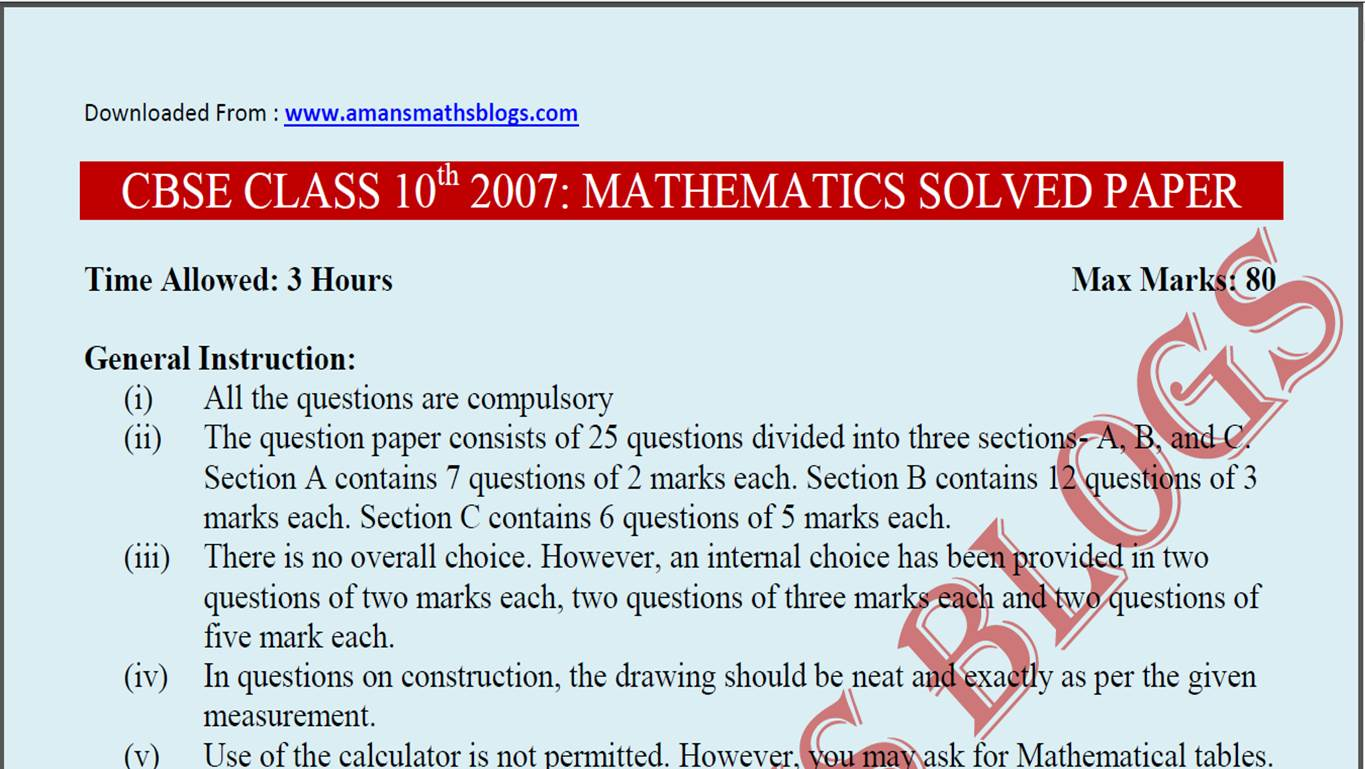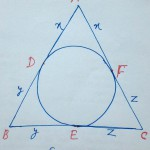Wednesday, January 22, 2020
Home > Latest Announcement > CBSE 10th 2007: MATHEMATICS SOLVED PAPER

# CBSE 10th 2007: MATHEMATICS SOLVED PAPER# Class 10 Maths: CBSE 2007 Solved Paper

In this post, you will get the CBSE Class 10 Maths 2007 Solved Papers. This is very important for the CBSE students who are appearing in CBSE Board Class 10 exam this year.

## CBSE CLASS 10th 2007: MATHEMATICS SOLVED PAPER

Time Allowed: 3 Hours                                                                                                    Max Marks: 80

General Instruction:

• 1. All the questions are compulsory. Get CBSE class 10 previous year question paper with solution.
• 2. The question paper consists of 25 questions divided into three sections- A, B, and C. Section A contains 7 questions of 2       marks each. Section B contains 12 questions of 3 marks each. Section C contains 6 questions of 5 marks each. CBSE Class 10 Maths 2007 Solved Papers.
• 3. There is no overall choice. However, an internal choice has been provided in two questions of two marks each, two questions     of three marks each and two questions of five mark each.
• 4. In questions on construction, the drawing should be neat and exactly as per the given measurement.
• 5. Use of the calculator is not permitted. However, you may ask for Mathematical tables.

Section A: Question No 1 to 7 carry 2 marks each.

Ques  : Find the GCD of the following polynomials 12x4 + 324x and 36x3 + 90x2 – 54x

Solution:  12x4 + 324x = 12x(x3 + 27) = 6 × 2x(x3 + (3)3) = 6 × 2x (x + 3)(x2 + 9 –3x)

36x3 + 90x2 – 54x = 18x(2x2 + 5x – 3) = 6 × 3x (2x2 + 6x – x – 3)

= 6 × 3x (2x (x+ 3) – 1(x + 3))

= 6 × 3x (x+ 3)(2x  – 1)

Thus, the GCD of the given polynomials is 6x (x + 3).

Ques  : Solve for x and y:andSolution: We are given the equations as…(1)…(2)

From (1) + (2), we getNow, putting the value of x in the equation (1), we getThus, the solution of the given equations are x = 2a and y = –2b.

OR

Solve for x and y:  31x + 29y = 33 and 29x + 31y = 27

Solution: We are given the equations as

31x + 29y = 33             …(1)

29x + 31y = 27             …(2)

From (1) + (2), we get

⇒31x + 29y + 29x + 31y = 33 + 27

⇒60x + 60y = 60

⇒60(x + y) = 60

⇒x + y = 1                   …(3)

From (1) – (2), we get

⇒31x + 29y – 29x – 31y = 33 – 27

⇒2x – 2y = 6

⇒2(x – y) = 6

⇒x – y = 3                   …(4)

From (3) + (4), we get

⇒2x = 4 x = 2

From (3) – (4), we get

⇒2y = –2 y = –1

Thus, we get x = 2 and y = –1

Ques : Find the sum of all the three digits whole numbers which are multiple of 7Solution: All the three-digits whole numbers are 100, 101, 102,…, 999.

Out of which, the multiples of 7 are 105, 112, 119, …, 994

Now,

⇒994 = 105 + (n – 1)(7)

⇒994 –105 = 7(n –1)

⇒889 = 7(n –1)⇒ n = 127 + 1 = 128

Thus, the required sum of the whole numbers which are multiples of 7 isQues : In the figure (1), PQ || AB and PR || AC. Prove that QR || BC.

Solution: Since PQ || AB in ∆OAB, we get…(1)           [ By Basic Proportionally Theorem ]

Since PR || AC in ∆OAC, we get…(2)           [ By Basic Proportionally Theorem ]

From the equations (1) and (2), we getQR | | BC             [ By Converse of Basic Proportionally Theorem ]

OR

In the figure (2) incircle of ∆ABC touches its sides AB, BC and CA at D, E and F respectively. If AB = AC,  prove that BE = ECSolution:

We know that the tangents drawn from an external point to the circle are equal in length. Thus, we getNow, we have    AB = AC ⇒ x + y = x + z ⇒ y = z ⇒ BE = EC.

Ques : If the mean of the following frequency distribution is 49, find the missing frequency p:

CLASSFREQUENCY
0-202
20-406
40-60P
60-805
80-1002

Solution:

ClassClass-Mark (x)Frequency
(f)
x×f
TOTAL15 + p730 + 50p
0-2010220
20-40306180
40-6050p50p
60-80705350
80-100902180

Now, we know that the mean of the grouped frequency isQues : A wrist watch is available for Rs. 1000 cash or Rs. 500 as cash down payment followed by three equal monthly installments of Rs. 180. Calculate the rate of interest charged under the installment plan.Solution: Here, we have

P = Rs. 1000,

A = Rs. (500 + 180 × 3) = Rs. (500 + 540) = Rs 1040

T = 3 Months =years

SI = A – P = Rs. (1040 – 1000) = Rs. 40. Thus, we need to find the rate of interest R = ?

We know thatTherefore, the rate of the interest is R = 16% per year

Ques : An unbiased die is tossed once. Find the probability of getting (i) A multiple of 2 or 3

(ii) A prime number greater than 2.

Solution:  In an unbiased die, we have {1,2,3,4,5,6} Thus, we have n(S) = 6

(i) A = multiple of 2 = {2,4,6} ⇒ n(A) = 3

B = multiple of 3 = {3,6}  ⇒ n(B) = 2

A ∩ B = multiple of both 2 and 3 = {6} ⇒ n(A ∩ B) = 1

Thus, the required probability of getting a multiple of 2 or 3 is(ii) A = A prime number greater than 2 = {3,5} n(A) = 2.

Thus, the required probability of getting a prime number greater than 2 iserror: Content is protected !!